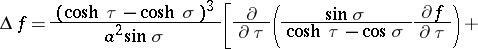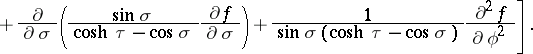# Bipolar coordinates

The numbersandwhich are connected with the Cartesian orthogonal coordinatesand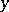by the formulaswhere. The coordinate lines are two families of circles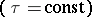with polesandand the (half-c)ircles orthogonal with these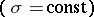.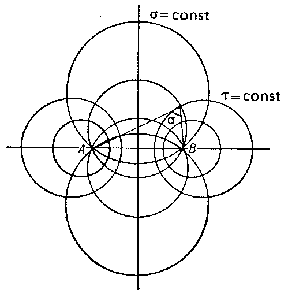Figure: b016470a

The Lamé coefficients are: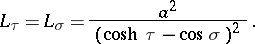The Laplace operator is: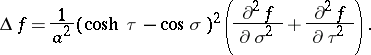Bipolar coordinates in space (bispherical coordinates) are the numbers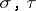and, which are connected with the orthogonal Cartesian coordinates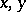andby the formulas:where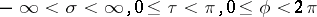. The coordinate surfaces are spheres (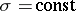), the surfaces obtained by rotation of arcs of circles (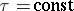) and half-planes passing through the-axis. The system of bipolar coordinates in space is formed by rotating the system of bipolar coordinates on the plane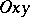around the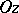-axis.

The Lamé coefficients are: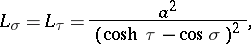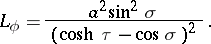The Laplace operator is: